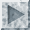## Background Information

If all faces of a polyhedron are (not necessarily congruent) regular polygons and all vertices are congruent, we call the polyhedron uniform. Regular polyhedra are, of course, also uniform. There are 18 convex uniform polyhedra, namely the five Platonic solids and thirteen Archimedean solids. There are also two families of prisms and antiprisms. One such solid exists for each regular n-gon, for n=2, 3, ... (the case n=2 is degenerate). Here are these twenty solids. We chose n=5 as representative of the prisms and antiprisms.
Platonic Solids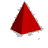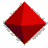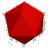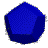Archimedian Solids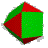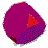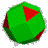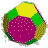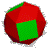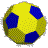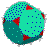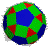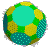Prisms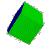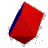If we drop the condition that the solids be convex we get many more shapes. First, we can allow non-convex polygons as faces. The non-convex regular polygons are the star polygons, such as the pentagram. The symbol n/d denotes a star n-gon obtained by connecting every dth vertex of an ordinary n-gon. We can also arrange the faces around a vertex in a non-convex way. With these additional figures we get four more regular solids, the Kepler-Poinsot polyhedra (see also the article by Nelson M. Blachmann [Blachmann93]). They are displayed here.
Kepler-Poinsot Solids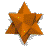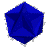Truncation consists of ``cutting off'' a vertex. For these polyhedra the cut figure is a regular polygon, since the vertex figure is regular. If we cut in such a way that the faces turn into new regular polygons with twice as many edges, we get another uniform polyhedron. If the cuts meet in the middle of the edges, we get yet another solid. The truncated small stellated dodecahedron is an ordinary dodecahedron, and the truncated great stellated dodecahedron turns into an icosahedron. The truncated-in-the-middle forms of the great icosahedron and the small stellated dodecahedron are the same, called the great icosidodecahedron, and so are the forms of the great dodecahedron and the great stellated dodecahedron, the dodecadodecahedron.
Truncated Kepler-Poinsot Solids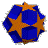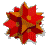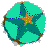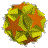The standard Mathematica package `Graphics/Polyhedra.m` contains an operation `Truncate[graphics3D, ratio]` that performs this operation on any polyhedron. Some of the Archimedian solids can be obtained in this way from the Platonic ones.

Here, we will not use these operations. Instead, we will find a way to compute the coordinates of any of the uniform polyhedra directly from their so-called Wythoff symbols. The list of all 75 uniform polyhedra (excluding the prisms and antiprisms) was first described by Coxeter et. al. [Coxeter53] and later shown to be complete by J. Skilling [Skilling75] based on a computer-generated enumeration of all possibilities.